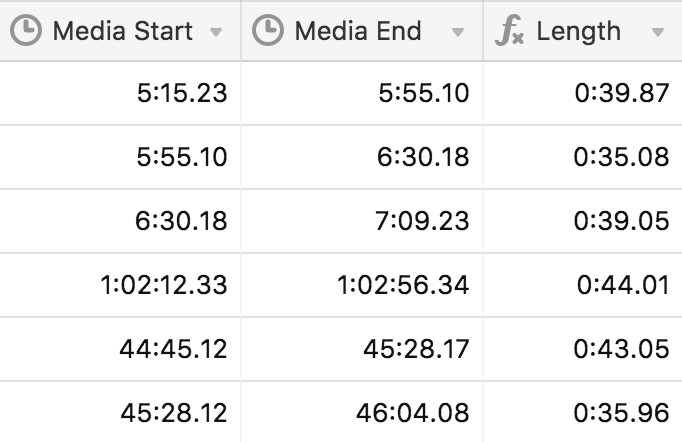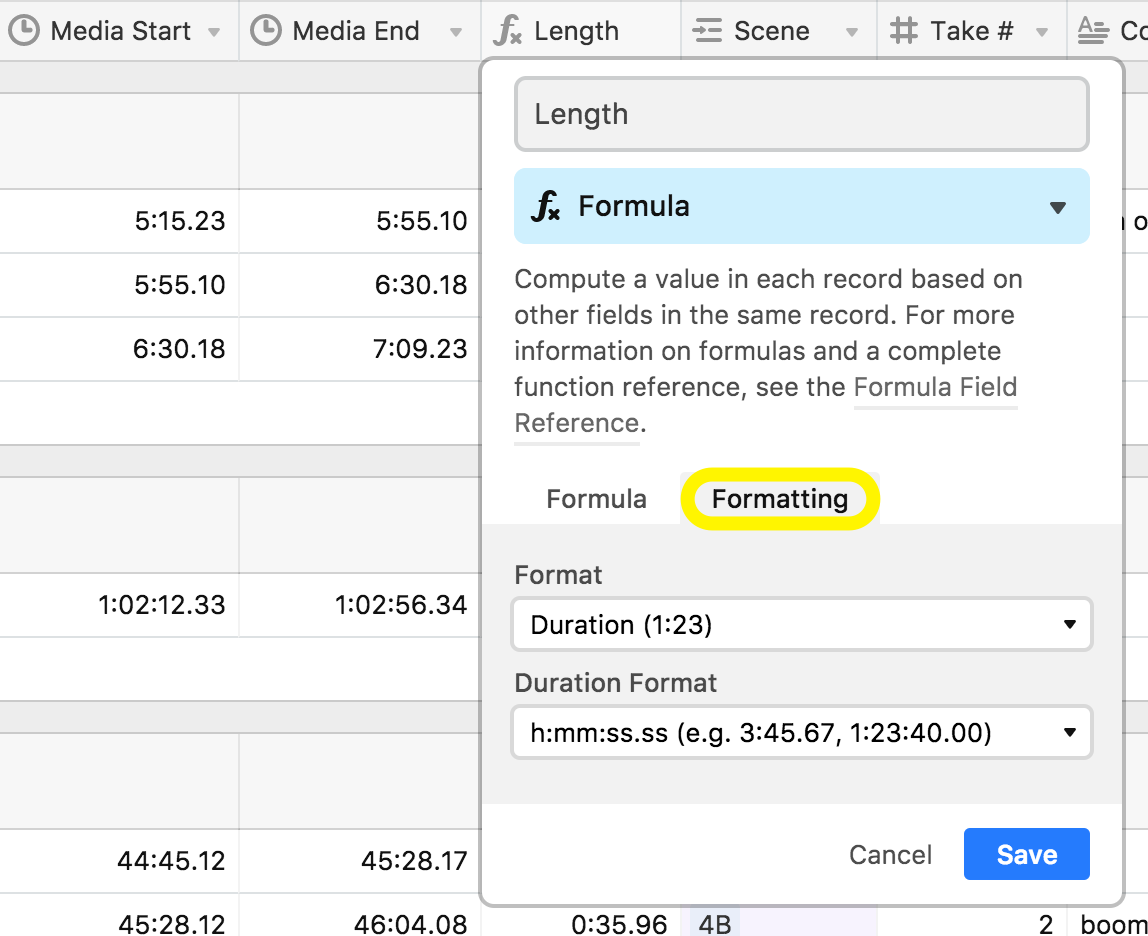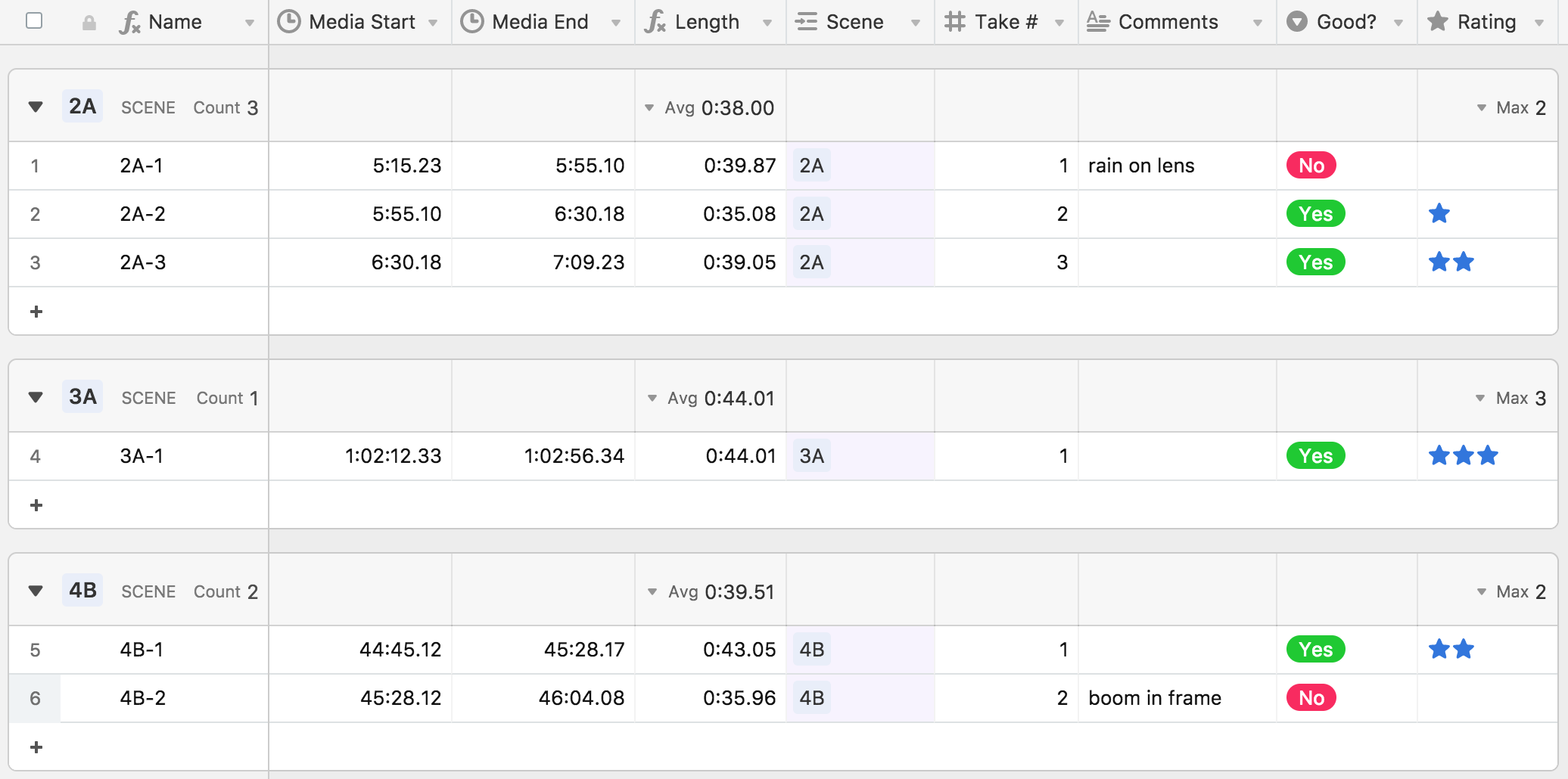• English
• Account
• API
• Integrations
• Enterprise
• Resources
Duration field type
• 01 Sep 2022
• Dark
Light

# Duration field type

• Dark
Light

Article Summary

The duration field type is a numeric field type intended for use with time durations measured in hours, minutes, seconds, or milliseconds. Depending on the format of the duration field, the values are either stored as an integer or decimal representing a number of seconds. (If you want to store a time duration measured in days, weeks, or other larger units, use a regular number field.)There are five formatting options available for duration fields.

• h:mm - for hours and minutes, e.g. 1:23
• h:mm:ss - for minutes and seconds, e.g. 3:45, or hours, minutes, and seconds, such as 1:23:40
• h:mm:ss.s - for minutes, seconds, and deciseconds, e.g. 3:45.6, or hours, minutes, seconds, and deciseconds, such as 1:23:40.0
• h:mm:ss.ss - for minutes, seconds, and centiseconds, e.g. 3:45.67, or hours, minutes, seconds, and centiseconds, such as 1:23:40.00
• h:mm:ss.sss - for minutes, seconds, and milliseconds, e.g. 3:45.678, or hours, minutes, seconds, and milliseconds, such as 1:23:40.000

NOTE

When formatting the output of the duration field any fractional values will round up to the next closest value. For example, if you select `h:mm` formatting, but then input `0.50`, the value duration outputted would round up to `0:01` since `0.50` represents a half of a second.

## Durations in computed fields

You can also format numeric formulas, lookups, or rollup fields as a duration—for example, multiplying a duration by a number, or rolling up the sum of a duration field from linked records. To format as a duration in a computed field, go to the field customization menu, click the Formatting tab, and select Duration from the Format dropdown. Once you do that, you can choose the specific Duration Format, e.g. h:mm:ss.ss.## Durations and date formulas

You can use simple math operators (like + for addition, - for subtraction, and so on) with durations. You can use a formula like `{End Time} - {Start time}` to compare durations (if you'd like your formula result as a duration make sure to format it as so).

You can also use durations with specific date/time formulas.

For example, you can use the `DATETIME_DIFF()` function to calculate the duration between two date fields with times and format the result as a duration. If you do this, do not specify a unit as the third parameter, e.g., use `DATETIME_DIFF({End Date}, {Start Date}),` not `DATETIME_DIFF({End Date}, {Start Date}, 'minutes')`. Then, be sure to click the Formatting tab in the field customization menu and select Duration from the Format dropdown.

You can also incorporate a duration field into a `DATEADD()` formula, for example, if you're trying to shift a time earlier or later with a formula. If you do this, please be sure to always provide 'seconds' as the `DATEADD()` unit, e.g. `DATEADD({Date field}, {Duration field}, '**seconds**')`. Note that when using DATEADD()you should make sure the timezone formatting is consistent between the date field you are using and the formula field returning it (e.g. they both use the same GMT time zone).

## FAQs

#### Why does my duration field's value increase when I use it in a formula?

Duration fields store values in seconds, regardless of the formatting option you choose to display visually.  You'll need to account for this in your formula by dividing by 60 if you want to calculate in minutes, 3600 (60 * 60) for hours, etc.

#### Why is my duration field not showing as a valid option for the y-axis of my chart?

While Duration fields are numeric fields, they are not valid options for the y-axis of a Chart extension. Fortunately, there's an easy solution. You can use a formula field to re-process your duration into a plain number, then use that formula field as your y-axis.  Here's an example formula to reformat your duration field in minutes:

`{Duration field} / 60`